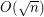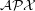# approximation algorithms

## Approximation ratio for k-outerplanar graphs ★★

Author(s): Bentz

Conjecture   Is the approximation ratio for the Maximum Edge Disjoint Paths (MaxEDP) or the Maximum Integer Multiflow problem (MaxIMF) bounded by a constant in-outerplanar graphs or tree-width graphs?

## Approximation Ratio for Maximum Edge Disjoint Paths problem ★★

Author(s): Bentz

Conjecture   Can the approximation ratiobe improved for the Maximum Edge Disjoint Paths problem (MaxEDP) in planar graphs or can an inapproximability result stronger than-hardness?